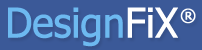Select Page

In a concrete component with at least three edges in a distance less than 1.5 times the embedment depth the resistance for conical concrete break-out, splitting and pry-out failure does not increase with increasing anchorage depth of the anchor. What is the reason for this illogical behaviour?

In a component with at least three edges in a distance of less than 1.5 times the anchorage depth, the break-out body and thus the idealized area Ac,N is truncated by these edges. The component will break at the position of the anchorage zone. Figure 1 illustrates this behavior using the example of a single anchor in a concrete prism (four edges).

Figure 1: Anchors with different embedment depths in a concrete prism

Therefore, the idealized fracture surface is not influenced by the anchorage depth of the anchor and the characteristic resistance for concrete break-out, splitting and pry-out failure is independent of hef. ETAG 001, Annex C, Section 5.2.2.4f considers this behaviour under ‘Special cases’. The actual anchorage depth hef is substituted by a fictive depth h’ef = max{hefcmax/ccr,N; hefsmax/scr,N}. Thereby, the idealized fracture surface just touches at least one edge and is not truncated at this side of the component. Figure 2 demonstrates this using an example with three edges with equal edge distances c.

Figure 2: Concrete component with three edges and equal edge distances c; definition of the real (hef) and fictive anchorage depth (h’ef)

[after: Eligehausen, R. Mallée, R. Silva, J. Anchorage in Concrete Construction. Ernst & Sohn, Berlin, 2006]

If this ‘Special case’ is not applied and the design is performed with the real embedment depth, then the resistance decreases with increasing anchorage depth. This is illogical.
DesignFiX applies this ‘Special case’ for applications with at least three component edges for the calculation of the characteristic resistance for conical concrete break-out, splitting and pry-out failure.## DesignFiX - Anchor Design